# FX 2D Transforms 1.0, Part 2: Language

## W3C Editor's Draft

This version:
Editors:
Anthony Grasso (Canon Inc.)
Dean Jackson (Apple Inc.)
Simon Fraser (Apple Inc.)
Authors:
The authors of this specification are the participants of the W3C FX Task Force.

## Abstract

Although originally designed for use in CSS and SVG, some aspects of this specification could be used in other environments, such as HTML styled with and XSL:FO.

This document defines the markup used by FX 2D Transforms.

## Status of This Document

This section describes the status of this document at the time of its publication. Other documents may supersede this document. The latest status of this document series is maintained at the W3C.

This document has been produced by the W3C FX Taskforce as part of the W3C Style Activity and Graphics Activity within the Interaction Domain.

We explicitly invite comments on this specification. Please send them to public-fx@w3.org (archives), the public email list for issues related to vector graphics on the Web. Acceptance of the archiving policy is requested automatically upon first post to either list. To subscribe to this list, please send an email to public-fx-request@w3.org with the word subscribe in the subject line.

The latest information regarding CSS patent disclosures and SVG patent disclosures to this document is available on the Web. As of this publication, the FX Taskforce are not aware of any royalty-bearing patents they believe to be essential to 2D Transforms.

Publication of this document does not imply endorsement by the W3C membership. A list of current W3C Recommendations and other technical documents can be found at http://www.w3.org/TR/. W3C publications may be updated, replaced, or obsoleted by other documents at any time. It is inappropriate to cite a W3C Working Draft as anything other than a work in progress.

## How to read this document and give feedback

This draft of XF 2D Transforms encapsulates the syntax and markup for the CSS 2D Transforms and SVG 2D Transforms languages. One of the goals is that this specification is to provide common behaviour and a common interface for both the CSS and SVG languages. Additionally, the specification can be re-used more easily by other specifications that want to have advanced 2D features. This specification introduces syntax that may not be backwards compatible with older SVG User Agents, and the use of this syntax should be accompanied by a fallback using the 'switch' element.

What should happen with CSS regarding backwards compatibility? Is that even an issue given this is the first transforms spec applicable to CSS?

The main purpose of this document is to encourage public feedback. The best way to give feedback is by sending an email to public-fx@w3.org. Please include some kind of keyword that identifies the area of the specification the comment is referring to in the subject line of your message (e.g "Section X.Y - Foo attribute values" or "2D Transforms Functionality"). If you have comments on multiple areas of this document, then it is probably best to split those comments into multiple messages.

The public are welcome to comment on any aspect in this document, but there are a few areas in which the FX Taskforce are explicitly requesting feedback. These areas are noted in place within this document. There is also a specific area related to the specification that is listed here:

## Contents

1. 1. Introduction
2. 2. Content Flow
3. 3. Coordinate system transformations
4. 4. Nested transformations
5. 5. The transform property
6. 6. The transform-origin property
7. 7. The Transformation Functions
8. 8. Transform Lists and Nested transformations
9. 9. Acknowledgments

## Introduction

The XF Taskforce will need to figure out how to merge the CSS 2D Transforms indroduction section and the SVG Transforms sections 7.1, 7.2, 7.3

## Content Flow

The transform property does not affect the flow of the content surrounding the transformed element. However, the value of the overflow area takes into account transformed elements. This behavior is similar to what happens when elements are translated via relative positioning. For elements in the CSS box model, if the value of the 'overflow' property is `scroll` or `auto`, scrollbars will appear as needed to see content that is transformed outside the visible area.

For elements in the CSS box model, specifying any value other than `none` for the 'transform' property results in the creation of both a stacking context and a containing block. The object acts as a containing block for fixed positioned descendants.

For both CSS elements in the CSS box model and SVG elements, specifying any value other than `none` for the 'transform' property establishes a new local coordinate system at the element that it is applied to. In SVG, this is equivalent to establishing a new user space at any element within an SVG document fragment.

## Coordinate system transformations

A new user space (i.e., a new current coordinate system) can be established by specifying a Transform Function using the 'transform' property on elements defined by the host language. The 'transform' property transform user space coordinates and lengths on sibling attributes on the given element and all of its descendants. Transformations can be nested, in which case the effect of the transformations are cumulative.

Mathematically, all transformations can be represented as 3x3 transformation matrices of the following form: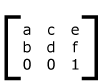Since only six values are used in the above 3x3 matrix, a transformation matrix is also expressed as a vector: [a b c d e f].

Transformations map coordinates and lengths from a new coordinate system into a previous coordinate system: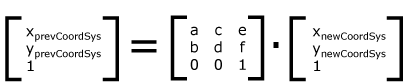Simple transformations are represented in matrix form as follows:

• Translation is equivalent to the matrix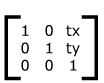or [1 0 0 1 tx ty], where tx and ty are the distances to translate coordinates in X and Y, respectively.

• Scaling is equivalent to the matrix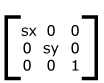or [sx 0 0 sy 0 0]. One unit in the X and Y directions in the new coordinate system equals sx and sy units in the previous coordinate system, respectively.

• Rotation about the origin is equivalent to the matrix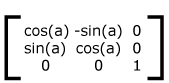or [cos(a) sin(a) -sin(a) cos(a) 0 0], which has the effect of rotating the coordinate system axes by angle a.

• A skew transformation along the x-axis is equivalent to the matrix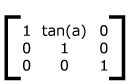or [1 0 tan(a) 1 0 0], which has the effect of skewing X coordinates by angle a.

• A skew transformation along the y-axis is equivalent to the matrix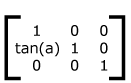or [1 tan(a) 0 1 0 0], which has the effect of skewing Y coordinates by angle a.

## The 'transform' property

A two-dimensional transformation is applied to an element through the '`transform`' property. This property contains a list of transform functions. The final transformation value for an element is obtained by performing a matrix concatenation of each entry in the list.

 Name: transform Value: none | [ ]* Initial: none Applies to CSS: block-level and inline-level elements Applies to SVG: container or graphics elements Inherited: no Percentages: refer to the size of the element's bounding box Media: visual Computed value: Same as specified value.

## The 'transform-origin' property

The '`transform-origin`' property establishes the origin of transformation for an element. This property is applied by first translating the element by the negated value of the property, then applying the element's transform, then translating by the property value. This effectively moves the desired transformation origin of the element to (0,0) in the local coordinate system, then applies the element's transform, then moves the element back to its original position.

 Name: transform-origin Value: [ [ | | left | center | right ] [ | | top | center | bottom ]? ] | [ [left | center | right ] || [ top | center | bottom ] ] Initial: auto Applies to CSS: block-level and inline-level elements Applies to SVG: container or graphics elements Inherited: no Percentages: refer to the size of the element's bounding box Media: visual Computed value: For the absolute value, otherwise a percentage

For elements in the CSS box model a value of auto for 'transform-origin' specifies the center (50%,50%) of the element.

For SVG elements a value of auto for 'transform-origin' specifies the origin (0,0) of the coordinate space that the element is in. Percentage values specified for a transform on an SVG element are relative to the bounding box of that element.

If only one value is specified, the second value is assumed to be '`center`'. If at least one value is not a keyword, then the first value represents the horizontal position and the second represents the vertical position. Negative <percentage> and <length> values are allowed.

If a single dimension of the element's bounding box is zero, then 'transform-origin' value will only effect the non-zero dimension of the element. If the both dimensions of the element's bounding box are zero, then the 'transform-origin' has no effect on the element.

## The Transformation Functions

The value of the transform property is a list of <transform-functions> applied in the order provided. The individual transform functions are separated by whitespace. The set of allowed transform functions is given below. In this list the type <translation-value> is defined as a <length> or <percentage> value, and the <angle> type is defined by CSS Values and Units.

none
Indicates that no tansform will be applied. The local coordinate system for the element remains unchanged and no new stacking context or containing block is establish.
identity
Specifies an identity transform. This is equivalent to specifying a transformataion matrix of [1 0 0 1 0 0].
matrix(<number>, <number>, <number>, <number>, <number>, <number>)
specifies a 2D transformation in the form of a transformation matrix of six values. matrix(a,b,c,d,e,f) is equivalent to applying the transformation matrix [a b c d e f].
translate(<translation-value>[,<translation-value>])
specifies a 2D translation by the vector [tx, ty], where tx is the first translation-value parameter and ty is the optional second translation-value parameter. If <ty> is not provided, ty has zero as a value.
translateX(<translation-value>)
specifies a translation by the given amount in the X direction.
translateY(<translation-value>)
specifies a translation by the given amount in the Y direction.
scale(<number>[, <number>])
specifies a 2D scale operation by the [sx,sy] scaling vector described by the 2 parameters. If the second parameter is not provided, it is takes a value equal to the first.
scaleX(<number>)
specifies a scale operation using the [sx,1] scaling vector, where sx is given as the parameter.
scaleY(<number>)
specifies a scale operation using the [1,sy] scaling vector, where sy is given as the parameter.
rotate(<angle> [,<cx>, <cy>])
specifies a 2D rotation by the angle specified in the parameter about the origin of the element. If optional parameters <cx> and <cy> are not supplied, the rotation is about the origin as defined by the transform-origin property. If optional parameters <cx> and <cy> are supplied, the rotation is about the point (cxcy) from the origin as defined by the transform-origin property. The operation represents the equivalent of the following specification: translate(<cx>, <cy>) rotate(<rotate-angle>) translate(-<cx>, -<cy>) .
skewX(<angle>)
specifies a skew transformation along the X axis by the given angle.
skewY(<angle>)
specifies a skew transformation along the Y axis by the given angle.

Move the element by 100 pixels in both the X and Y directions.

```    CSS: div {transform: translate(100px, 100px);}

SVG: <rect transform="translate(100 100)"/>
```
Without transform Translation transform applied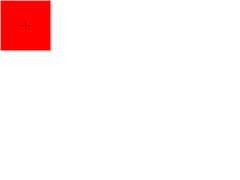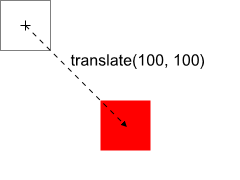Scale the element by a factor of 2 in both the X and Y directions.

```    CSS: div {transform: scale(2, 2);}

SVG: <rect transform="scale(2 2)"/>
```
Without transform Scale transform applied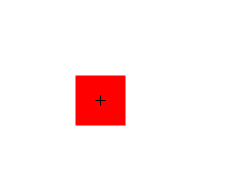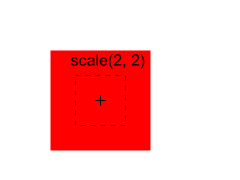Rotate the element by 45 degrees.

```    CSS: div {transform: rotate(45deg);}

SVG: <rect transform="rotate(45)"/>
```
Without transform Rotate transform applied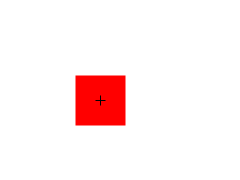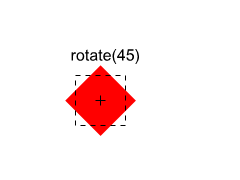## Transform Lists and Nested Transformations

Transformations can be nested to any level and are cumulative. That is, elements establish their local coordinate system within the coordinate system of their parent. A nested '`transform`' property effectively accumulates all the tranform properties of its ancestors. The accumulated transformation for an element is derived by post-multiplying the subsequent transformation properties of its ancestors onto the transformation specified for the element. The accumulation of these transforms defines a current transformation matrix (CTM) for the element.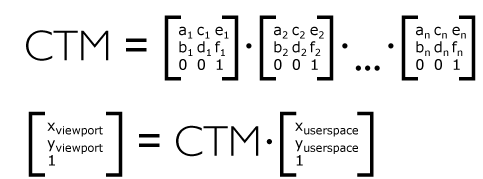```
``` ``` ```

The above syntax would transform an element as illustrated below. The object is moved by 100px in both the X and Y direction from its original position. Next, its size is scaled by 150% in both the X and Y direction. Lastly, the object is rotated 45 degrees clockwise about the Z axis. Note that the element has a default transform origin of "50% 50%", meaning the scale and rotation transforms operate about the center of the object.

If a list of transforms is provided, then the net effect is as if each transform had been specified separately in the order provided. For example,

```<div style="transform:translate(-10px,-20px) scale(2) rotate(45deg) translate(5px,10px)"/>
```

is functionally equivalent to:

```<div style="transform:translate(-10px,-20px)">
<div style="transform:scale(2)">
<div style="transform:rotate(45deg)">
<div style="transform:translate(5px,10px)">
</div>
</div>
</div>
</div>
```

## Transitions and animations between transform values

When animating or transitioning the value of a transform property the rules described below are applied. The '`from`' transform is the transform at the start of the transition or current keyframe. The '`end`' transform is the transform at the end of the transition or current keyframe.

• If the '`from`' and '`to`' transforms are both single functions of the same type:
• For `identity`, `translate`, `translateX`, `translateY`, `scale`, `scaleX`, `scaleY`, `rotate`, `skewX` and `skewY` transform functions:
• the individual components of the function are interpolated numerically.
• For matrix:
• the matrix is decomposed using the method described by unmatrix [SPENCER] into separate translation, scale, rotation and skew matrices, then each decomposed matrix is interpolated numerically, and finally combined in order to produce a resulting 3x2 matrix.
• If both the '`from`' and '`to`' transforms are `identity` or `none`:
• There is no interpolation necessary
• If one of the '`from`' or '`to`' transforms is `none`:
• The `none` is replaced by the `identity` transform function from the transform function list.
• If both the '`from`' and '`to`' transforms have the same number of transform functions and corresponding functions in each transform list are of the same type:
• Each transform function is animated with its corresponding destination function in isolation using the rules described above. The individual values are then applied as a list to produce resulting transform value.
• Otherwise:
• The transform function lists are each converted into the equivalent matrix value and animation proceeds using the rule for a single function above.

In some cases, an animation might cause a transformation matrix to be singular or non-invertible. For example, an animation in which scale moves from 1 to -1. At the time when the matrix is in such a state, the transformed element is not rendered.

## Matrix decomposition for animation

When interpolating between 2 matrices, each is decomposed into the corresponding translation, rotation, scale, skew, and perspective values. Not all matrices can be accurately described by these values. Those that can't are decomposed into the most accurate representation possible, using the technique below. This technique is taken from The "unmatrix" method in "Graphics Gems II, edited by Jim Arvo". The pseudocode below works on a 4x4 homogeneous matrix. A 3x2 2D matrix is therefore first converted to 4x4 homogeneous form.

```  Input: matrix       ; a 4x4 matrix
Output: translation ; a 3 component vector
rotation    ; Euler angles, represented as a 3 component vector
scale       ; a 3 component vector
skew        ; skew factors XY,XZ,YZ represented as a 3 component vector
perspective ; a 4 component vector
Returns false if the matrix cannot be decomposed, true if it can

Supporting functions (point is a 3 component vector, matrix is a 4x4 matrix):
float  determinant(matrix)          returns the 4x4 determinant of the matrix
matrix inverse(matrix)              returns the inverse of the passed matrix
matrix transpose(matrix)            returns the transpose of the passed matrix
point  multVecMatrix(point, matrix) multiplies the passed point by the passed matrix
and returns the transformed point
float  length(point)                returns the length of the passed vector
point  normalize(point)             normalizes the length of the passed point to 1
float  dot(point, point)            returns the dot product of the passed points
float  cos(float)                   returns the cosine of the passed angle in radians
float  asin(float)                  returns the arcsine in radians of the passed value
float  atan2(float y, float x)      returns the principal value of the arc tangent of
y/x, using the signs of both arguments to determine
the quadrant of the return value

Decomposition also makes use of the following function:
point combine(point a, point b, float ascl, float bscl)
result = (ascl * a) + (bscl * b)
result = (ascl * a) + (bscl * b)
result = (ascl * a) + (bscl * b)
return result

// Normalize the matrix.
if (matrix == 0)
return false

for (i = 0; i < 4; i++)
for (j = 0; j < 4; j++)
matrix[i][j] /= matrix

// perspectiveMatrix is used to solve for perspective, but it also provides
// an easy way to test for singularity of the upper 3x3 component.
perspectiveMatrix = matrix

for (i = 0; i < 3; i++)
perspectiveMatrix[i] = 0

perspectiveMatrix = 1

if (determinant(perspectiveMatrix) == 0)
return false

// First, isolate perspective.
if (matrix != 0 || matrix != 0 || matrix != 0)
// rightHandSide is the right hand side of the equation.
rightHandSide = matrix;
rightHandSide = matrix;
rightHandSide = matrix;
rightHandSide = matrix;

// Solve the equation by inverting perspectiveMatrix and multiplying
// rightHandSide by the inverse.
inversePerspectiveMatrix = inverse(perspectiveMatrix)
transposedInversePerspectiveMatrix = transposeMatrix4(inversePerspectiveMatrix)
perspective = multVecMatrix(rightHandSide, transposedInversePerspectiveMatrix)

// Clear the perspective partition
matrix = matrix = matrix = 0
matrix = 1
else
// No perspective.
perspective = perspective = perspective = 0
perspective = 1

// Next take care of translation
translate = matrix
matrix = 0
translate = matrix
matrix = 0
translate = matrix
matrix = 0

// Now get scale and shear. 'row' is a 3 element array of 3 component vectors
for (i = 0; i < 3; i++)
row[i] = matrix[i]
row[i] = matrix[i]
row[i] = matrix[i]

// Compute X scale factor and normalize first row.
scale = length(row)
row = normalize(row)

// Compute XY shear factor and make 2nd row orthogonal to 1st.
skew = dot(row, row)
row = combine(row, row, 1.0, -skew)

// Now, compute Y scale and normalize 2nd row.
scale = length(row)
row = normalize(row)
skew /= scale;

// Compute XZ and YZ shears, orthogonalize 3rd row
skew = dot(row, row)
row = combine(row, row, 1.0, -skew)
skew = dot(row, row)
row = combine(row, row, 1.0, -skew)

// Next, get Z scale and normalize 3rd row.
scale = length(row)
row = normalize(row)
skew /= scale
skew /= scale

// At this point, the matrix (in rows) is orthonormal.
// Check for a coordinate system flip.  If the determinant
// is -1, then negate the matrix and the scaling factors.
pdum3 = cross(row, row)
if (dot(row, pdum3) < 0)
for (i = 0; i < 3; i++) {
scale *= -1;
row[i] *= -1
row[i] *= -1
row[i] *= -1

// Now, get the rotations ou
rotate = asin(-row);
if (cos(rotate) != 0)
rotate = atan2(row, row);
rotate = atan2(row, row);
else
rotate = atan2(-row, row);
rotate = 0;

return true;
```

Each component of each returned value is linearly interpolated with the corresponding component of the other matrix. The resulting components are then recomposed into a final matrix as though combining the following transform functions:

```        matrix3d(1,0,0,0, 0,1,0,0, 0,0,1,0, perspective, perspective, perspective, perspective)
translate3d(translation, translation, translation)
rotateX(rotation) rotateY(rotation) rotateZ(rotation)
matrix3d(1,0,0,0, 0,1,0,0, 0,skew,1,0, 0,0,0,1)
matrix3d(1,0,0,0, 0,1,0,0, skew,0,1,0, 0,0,0,1)
matrix3d(1,0,0,0, skew,1,0,0, 0,0,1,0, 0,0,0,1)
scale3d(scale, scale, scale)
```

## RelaxNG Schema for FX 2D Transforms 1.0

The schema for FX 2D Transforms 1.0 is written in RelaxNG [RelaxNG], a namespace-aware schema language that uses the datatypes from XML Schema Part 2 [Schema2]. This allows namespaces and modularity to be much more naturally expressed than using DTD syntax. The RelaxNG schema for SVG Filter 1.2 may be imported by other RelaxNG schemas, or combined with other schemas in other languages into a multi-namespace, multi-grammar schema using Namespace-based Validation Dispatching Language [NVDL].

Unlike a DTD, the schema used for validation is not hardcoded into the document instance. There is no equivalent to the DOCTYPE declaration. Simply point your editor or other validation tool to the IRI of the schema (or your local cached copy, as you prefer).

The RNG is under construction, and only the individual RNG snippets are available at this time. They have not yet been integrated into a functional schema. The individual RNG files are available here.

## DOM interfaces

The following interfaces are defined below: Point.

These should probably move into the CSSOM View Module eventually.

Include a class that similar to CSSMatrix/SVGTransform here.

### Interface Point

The Point interface contains X and Y coordinates for a point on a 2D plane.

"Point" needs a prefix to avoid conflicting with lots of existing JavaScript. How does this relate to SVGPoint? What's a good name: WebPoint? ClientPoint (even though it's not always in client coordinates)? CSSPoint? We should probably say something about units here too, and maybe even add a section on coordinate spaces in CSS and SVG.

IDL Definition
```interface Point {
};```

Attributes
The X coordinate of the point.
The Y coordinate of the point.

### Extensions to the Element Interface

These extensions so the Element interface are implemented by all SVG and HTML elements, and provide the author a way to convert points between the coordinate spaces of different elements, taking transforms into account.

In CSS, the local coordinate system of an element is defined in CSS pixels, with the origin at the top, left corner of the border box, with positive values down and to the right.

What is the best behavior for inline elements, that may be split over multiple lines?

In CSS, the client coordinate system is defined by the CSS viewport. [Need Terminology section.]

In SVG, the local coordinate system is defined by ... [WRITE ME].

In SVG, the client coordinate system is defined by ... [WRITE ME].

IDL Definition
```[Supplemental] interface Element : Element {
Point clientToElement(Point p);
Point elementToClient(Point p);
};```

Methods
Point clientToLocalPoint(Point point)
Convert the given point from Client coordinates into the local coordinate space of the element.
Point localToClientPoint(Point point)
Convert the given point from the local coordinate space of this element into Client coordinates.

### Interface RandomInterface

The RandomInterface interface corresponds to the 'RandomInterface' element.

IDL Definition
```interface RandomInterface : SVGElement {
};```

Attributes
Corresponds to attribute bar on the given 'Random' element.

## References

### Normative References

[CSS21]
Cascading Style Sheets Level 2 Revision 1 (CSS 2.1) Specification, B. Bos, T. Çelik, I. Hickson, H. Wium Lie, eds. World Wide Web Consortium, 8 September 2009.
This edition of CSS 2.1 is http://www.w3.org/TR/2009/CR-CSS2-20090908/
The latest edition of SVG CSS 2.1 is available at http://www.w3.org/TR/CSS21/.
[RFC2119]
Key words for use in RFCs to Indicate Requirement Levels, S. Bradner, March 1997.
Available at http://tools.ietf.org/html/rfc2119.
[SVG11]
Scalable Vector Graphics (SVG) 1.1, J. Ferraiolo, 藤沢 淳 (Fujisawa Jun), D. Jackson, eds. World Wide Web Consortium, 14 January 2003.
This edition of SVG 1.1 is http://www.w3.org/TR/2003/REC-SVG11-20030114/.
The latest edition of SVG 1.1 is available at http://www.w3.org/TR/SVG11/.
[SVGT12]
Scalable Vector Graphics (SVG) Tiny 1.2, O. Andersson, R. Berjon, E. Dahlström, A. Emmons, J. Ferraiolo, A. Grasso, V. Hardy, S. Hayman, D. Jackson, C. Lilley, C. McCormack, A. Neumann, C. Northway, A. Quint, N. Ramani, D. Schepers, A. Shellshear, eds. World Wide Web Consortium, 22 December 2008.
This edition of SVG Tiny 1.2 is http://www.w3.org/TR/2008/REC-SVGTiny12-20081222/.
The latest edition of SVG Tiny 1.2 is available at http://www.w3.org/TR/SVGTiny12/.

### Informative References

[NVDL]
Information Technology — Document Schema Definition Languages (DSDL) — Part 4: Namespace-based Validation Dispatching Language: ISO/IEC FDIS 19757-4:2005(E), International Organization for Standardization, December 2005.
Available at http://www.jtc1sc34.org/repository/0694.pdf.
[SCHEMA2]
XML Schema Part 2: Datatypes Second Edition. P. Biron, A. Malhotra, eds. World Wide Web Consortium, 28 October 2004. (See also Processing XML 1.1 documents with XML Schema 1.0 processors [XML11-SCHEMA].)
This edition of XML Schema Part 2 is http://www.w3.org/TR/2004/REC-xmlschema-2-20041028/.
The latest edition of XML Schema Part 2 is available at http://www.w3.org/TR/xmlschema-2/.
[SPENCER]
Decomposing a Matrix Into Simple Transformations . Thomas, Spencer W. p. 320-323, code: p. 599-602, unmatrix.c.

### Acknowledgments

The editors would like to acknowledge and thank the following people for substantive aid with this specification: .